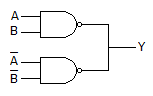# Electronics and Communication Engineering - Digital Electronics

16.

A pulse stretcher is same as a

 A. free running multivibrator B. bistable multivibrator C. monostable multivibrator D. latch

Explanation:

No answer description available for this question. Let us discuss.

17.

Binary multiplication 1 x 0 =

 A. 1 B. 0 C. 10 D. 11

Explanation:

No answer description available for this question. Let us discuss.

18.

The minimum decimal equivalent of the number 11C0 is

 A. 183 B. 194 C. 268 D. 269

Explanation:

No answer description available for this question. Let us discuss.

19.

The circuit of the given figure performsA. OR function B. AND function C. exclusive OR function D. exclusive NOR function

Explanation:

No answer description available for this question. Let us discuss.

20.

The hex number EB16 represents binary number of

 A. 11101011 B. 10010110 C. 10101100 D. 11101011